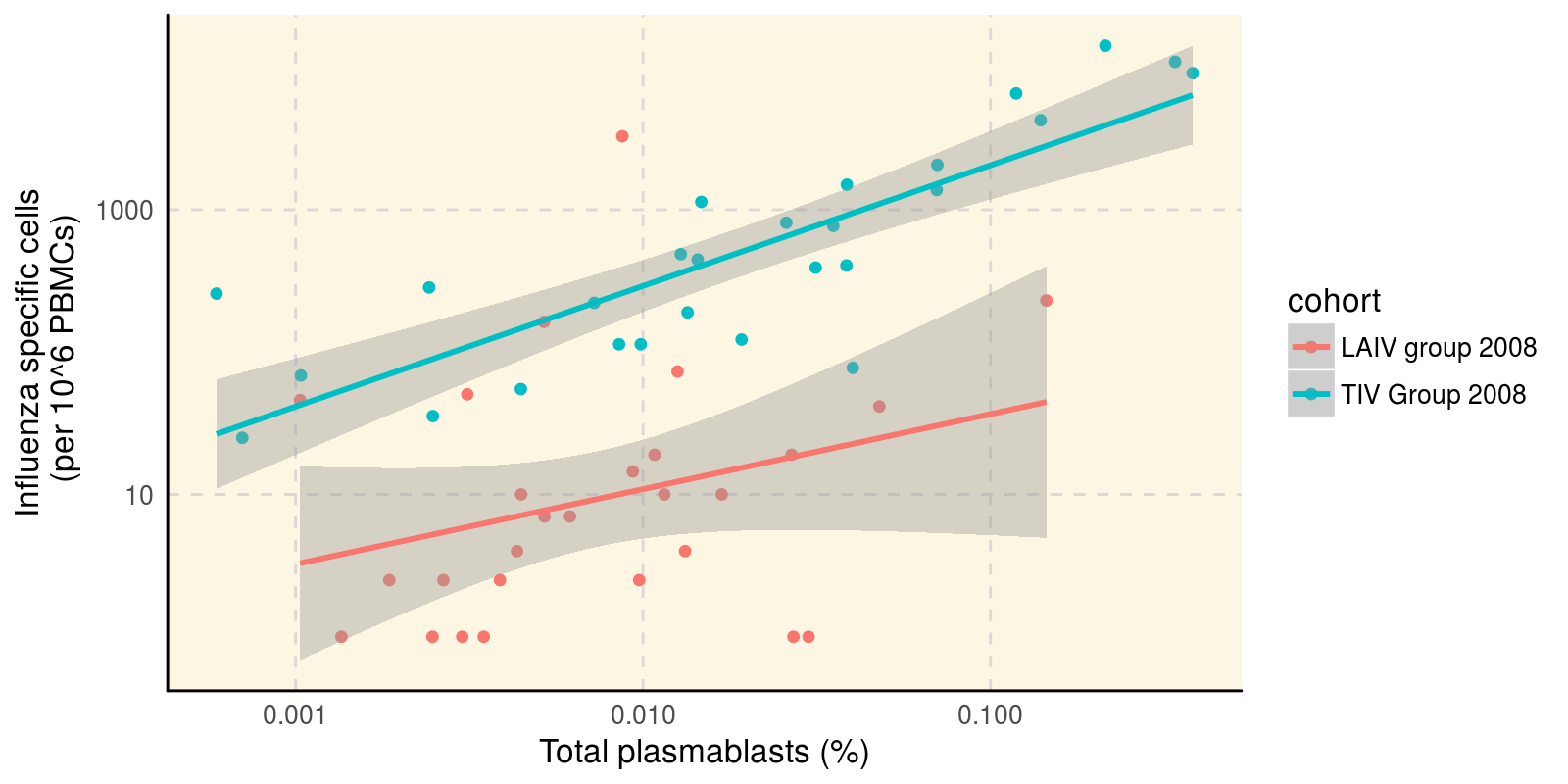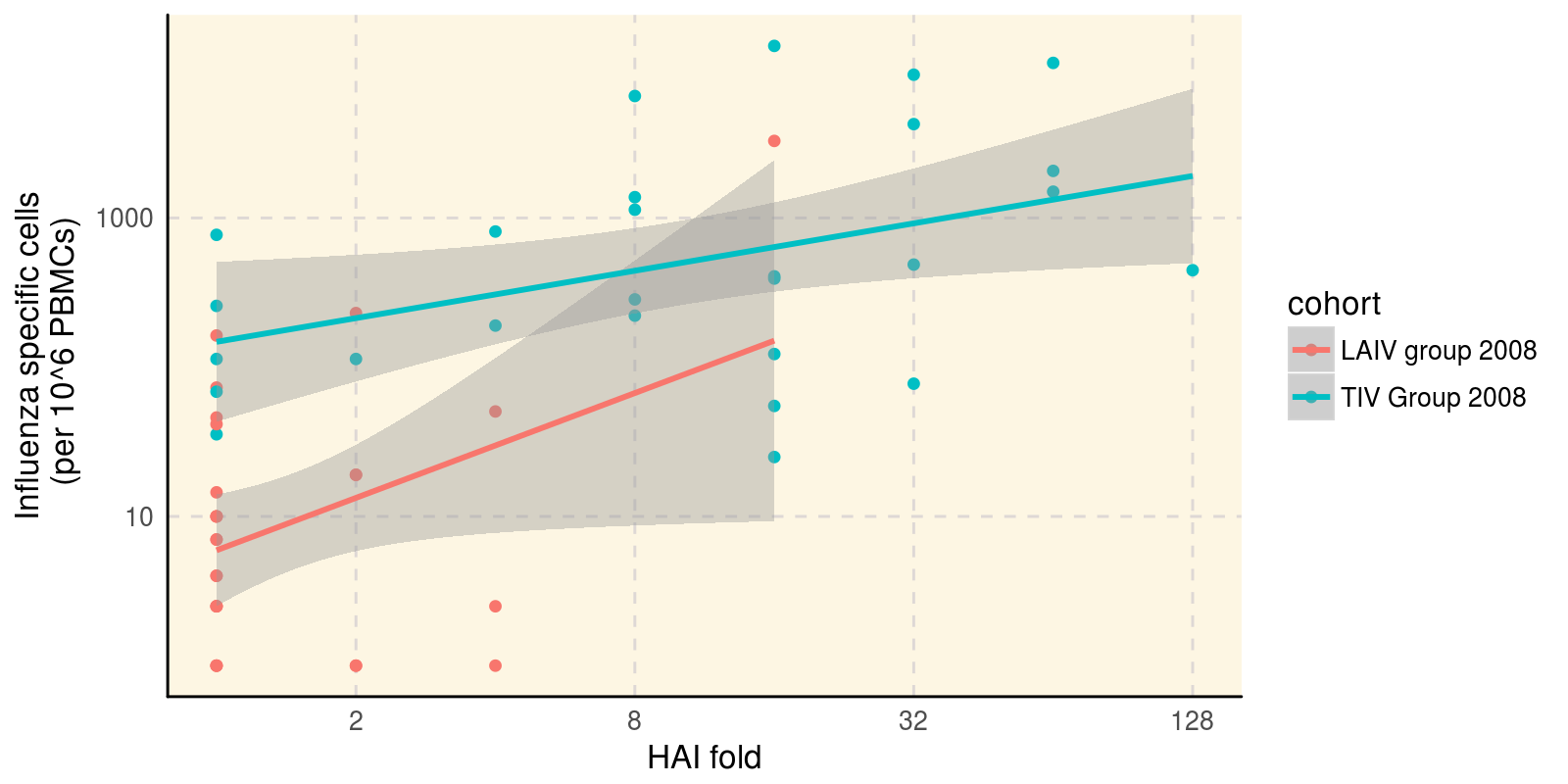# Correlating HAI with flow cytometry and ELISPOT results in SDY269

#### 2018-02-13

ImmuneSpaceR code produces consistent results, regardless of whether it is being executed from a module or UI based report on the server or on a local machine. This vignette reproduces a report available on www.immunespace.org using the same code.

### Summary

This report investigate the association between the number influenza-specific cells measured by ELISPOT measured at day 7 with the number of plasmablast measured by flow cytometry and day 7 and the HAI response measured at day 28 (log-fold day28/day0). It basically reproduces Figure 1 d-e) of Nakaya et al. (2011) published as part of the original study.

#### Load ImmuneSpaceR and other libraries

library(ImmuneSpaceR)
library(ggplot2)
library(data.table)

#### Connect to the study and get datasets

study <- CreateConnection(c("SDY269"))
dt_hai <- study$getDataset("hai", reload=TRUE) dt_fcs <- study$getDataset("fcs_analyzed_result", reload=TRUE)
dt_elispot <- study\$getDataset("elispot", reload=TRUE)

#### Transform data

# Compute max fold change for HAI, and remove time zero
dt_hai <- dt_hai[,hai_response:=value_reported/value_reported[study_time_collected==0],
by="virus,cohort,participant_id"][study_time_collected==28]
dt_hai <- dt_hai[,list(hai_response=max(hai_response)),by="cohort,participant_id"]

# Define variable for ELISPOT, keep only the IgG class
dt_elispot <- dt_elispot[,elispot_response:=spot_number_reported+1][study_time_collected==7 & analyte=="IgG"]
# Compute % plasmablasts
dt_fcs <- dt_fcs[,fcs_response:=(as.double(population_cell_number)+1) /
as.double(base_parent_population)][study_time_collected==7]

#### Merge data and phenodata

# Let's key the different datasets
setkeyv(dt_hai, c("participant_id"))
setkeyv(dt_fcs, c("participant_id"))
setkeyv(dt_elispot, c("participant_id"))
dt_all <- dt_hai[dt_fcs, nomatch=0][dt_elispot, nomatch=0]

The figure below shows the absolute number of plasmablast cells measured by flow cytometry vs. the number of frequency of influenza-specific cells measured by ELISPOT.

ggplot(dt_all, aes(x=as.double(fcs_response), y=elispot_response, color=cohort)) +
geom_point() + scale_y_log10() + scale_x_log10() + geom_smooth(method="lm") +
xlab("Total plasmablasts (%)") + ylab("Influenza specific cells\n (per 10^6 PBMCs)") +
theme_IS()The figure below shows the HAI fold increase over baseline vs. the number of frequency of influenza-specific cells measured by ELISPOT.

ggplot(dt_all, aes(x=as.double(hai_response), y=elispot_response, color=cohort)) +
geom_point() + scale_x_continuous(trans="log2") + scale_y_log10() +
geom_smooth(method="lm") + xlab("HAI fold") +
ylab("Influenza specific cells\n (per 10^6 PBMCs)") + theme_IS()In each case, we observe good correlations between the different responses, at least for the TIV cohort.